# Chemistry Topic-Wise Test - 8

## 31 Questions MCQ Test NEET Mock Test Series & Past Year Papers | Chemistry Topic-Wise Test - 8

Description
This mock test of Chemistry Topic-Wise Test - 8 for NEET helps you for every NEET entrance exam. This contains 31 Multiple Choice Questions for NEET Chemistry Topic-Wise Test - 8 (mcq) to study with solutions a complete question bank. The solved questions answers in this Chemistry Topic-Wise Test - 8 quiz give you a good mix of easy questions and tough questions. NEET students definitely take this Chemistry Topic-Wise Test - 8 exercise for a better result in the exam. You can find other Chemistry Topic-Wise Test - 8 extra questions, long questions & short questions for NEET on EduRev as well by searching above.
QUESTION: 1

Solution:
QUESTION: 2

Solution:
QUESTION: 3

### The moleucular weight of NaCl (degree of dissociation= x) determined by the osmotic pressure method, is found to be different from its actual molecular wieght (M). Which of the following relationship is correct ?

Solution:
QUESTION: 4
Phenol associates in benzene to produce double moleucles. To what degree phenol assicates if van’t Hoff factor is 0.54 ?
Solution:
QUESTION: 5
Dry air is bubbled successively through (i) a solution,(ii) its solvant, and (iii) through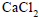The lowering of vapour pressure of the solvant due to the addition
of solute is equal to
Solution:
QUESTION: 6
At what temperature does an aqueous solution containing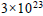molecules of a noneelectrolyte substance in 250g of water freeze ?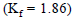Solution:
QUESTION: 7
The vapour pressure of a solvent decreased by 10mmHg when a nonvolatile solute was added to the solvent. The mole fraction of the solute in the solution
is 0.2. What would be the mole fraction of the solvent if the decrease in the vapour pressure is to be 20 mm Hg
Solution:
QUESTION: 8
Which of the following will not give iodoform test
Solution:
QUESTION: 9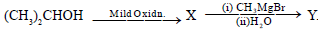Here Y is

Solution:
QUESTION: 10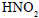forms an alcohol on reaction with
Solution:
QUESTION: 11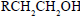can be converted into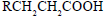by the following sequence of steps
Solution:
QUESTION: 12
Alkene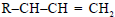reacts with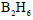in the presence of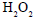to give
Solution:
QUESTION: 13
Vapours of an alcohol were passed over red hot copper. It gave an olefin. The alcohol is
Solution:
QUESTION: 14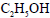cannot be dried by andhydrousbecause
Solution:
QUESTION: 15

Alcohol can be obtained by all methods except

Solution:

Solution :

C2H5MgBr + H2O = Mg(OH)Br + C2H6(Ethane)

QUESTION: 16

Increasing order of acid strength among tertiarybutanol, isopropanol and ehanol is

Solution:

Increase in R increases the e+ve of the element, so acidic strength decreases as R group increase in the compound. So acidic strength is in the order of Ethanol > Isopropanol > Tertiary Butanol

Hence B

QUESTION: 17
How many isomeric alcohols are possible with formula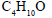Solution:
QUESTION: 18

Iodoform gives a precipitate with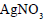on heating with but chloroform does not because

Solution:
QUESTION: 19
Chlorobenzene on fusing with solid NaOH gives
Solution:
QUESTION: 20
For the reactionthe order of reactivity is
Solution:
QUESTION: 21
Benzyl alcohol on reaction with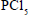gives
Solution:
QUESTION: 22
Methyl bromide and Ethyl bromide is mixed with equal proportions and the mixture is treated with metallic sodium. Which of the following is not expected to form
Solution:
QUESTION: 23
Aryl halides are less reactive towards neucliophilic substitution reactions as compared to alkyl halides due to
Solution:
QUESTION: 24

Propene is allowed to react with HI. The product (A) is then treated with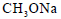to give a new product (B)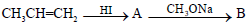Solution:
QUESTION: 25
The reaction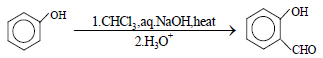is an example of
Solution:
QUESTION: 26

Which of the following statements about phenol is correct?

Solution:
QUESTION: 27
The utility of the polymers in various fields is due to their mechanical properties like tensile strength , elasticity, toughness etc. These properties mainly depend upon intermolecular forces like van der Waal’s forces and hydrogen bonding operating in polymer molecules. Polymers have been classified on this basis,e.g.(1) Elastomers (2) Thermoplacstics (4)Thermosetting. Hence
Vulcanized rubber is an example of
Solution:
QUESTION: 28
The utility of the polymers in various fields is due to their mechanical properties like tensile strength , elasticity, toughness etc.These properties mainly depend upon intermolecular forces like van der Waal’s forces and hydrogen bonding operating in polymer molecules. Polymers have been classified on this
basis,e.g.(1) Elastomers (2) Thermoplacstics (4)Thermosetting. Hence
The molecular forces of attraction are weakest in
Solution:
QUESTION: 29
The utility of the polymers in various fields is due to their mechanical properties like tensile strength , elasticity, toughness etc.These properties mainly depend upon intermolecular forces like van der Waal’s forces and hydrogen bonding operating in polymer molecules. Polymers have been classified on this
basis,e.g.(1) Elastomers (2) Thermoplacstics (4)Thermosetting. Hence
Which of the following have usually a linear structure?
Solution:
QUESTION: 30
The utility of the polymers in various fields is due to their mechanical properties like tensile strength , elasticity, toughness etc.These properties mainly depend upon intermolecular forces like van der Waal’s forces and hydrogen bonding operating in polymer molecules. Polymers have been classified on this
basis,e.g.(1) Elastomers (2) Thermoplacstics (4)Thermosetting. Hence
Nylon-6 which is fibre is made from
Solution:
QUESTION: 31
The utility of the polymers in various fields is due to their mechanical properties like tensile strength , elasticity, toughness etc.These properties mainly depend upon intermolecular forces like van der Waal’s forces and hydrogen bonding operating in polymer molecules. Polymers have been classified on this
basis,e.g.(1) Elastomers (2) Thermoplacstics (4)Thermosetting. Hence
Which of the following is hard

Solution: